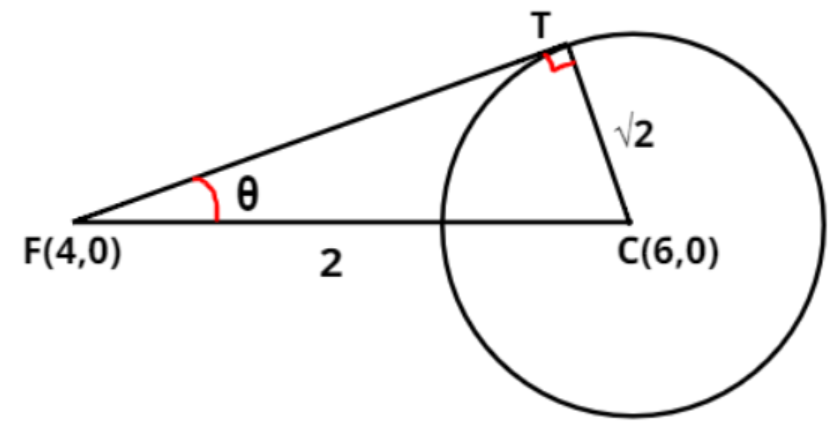Courses
Courses for Kids
Free study material
Free LIVE classes
MoreLIVE
Join Vedantu’s FREE Mastercalss

# The focal chord to ${y^2} = 16x$ is tangent to ${\left( {x - 6} \right)^2} + {y^2} = 2$, then the possible value of the slope of this chord areA). $\left( { - 1,1} \right)$ B). $\left( { - 2,2} \right)$ C). $\left( { - 2,\dfrac{1}{2}} \right)$ D). $\left( {2, - \dfrac{1}{2}} \right)$Verified
364.5k+ views
Hint: Here, we will proceed by comparing the given equations with general equations of parabola and circle.

The given equation of the parabola is ${y^2} = 16x{\text{ }} \to {\text{(1)}}$
The general form of an upward parabola having focus ${\text{F}}\left( {a,0} \right)$ is ${y^2} = 4ax{\text{ }} \to {\text{(2)}}$
On comparing above equations (1) and (2), we get
$4a = 16 \Rightarrow a = 4$
Hence, focus of the given parabola is ${\text{F}}\left( {4,0} \right)$
It is also given that the focal chord of the given parabola is tangent to the circle ${\left( {x - 6} \right)^2} + {y^2} = 2 \Rightarrow {\left( {x - 6} \right)^2} + {\left( {y - 0} \right)^2} = {\left( {\sqrt 2 } \right)^2}{\text{ }} \to {\text{(3)}}$.
Since, the general equation of a circle with centre coordinate as ${\text{C}}\left( {{x_1},{y_1}} \right)$ and radius as $r$ is given by ${\left( {x - {x_1}} \right)^2} + {\left( {y - {y_1}} \right)^2} = {r^2}{\text{ }} \to {\text{(4)}}$
On comparing equations (3) and (4), we get
The centre coordinate of the given circle is ${\text{C}}\left( {6,0} \right)$ and radius is $\sqrt 2$.
Since we know that distance between two points ${\text{A}}\left( {a,b} \right)$ and ${\text{B}}\left( {c,d} \right)$ is given by distance formula which is $d = \sqrt {{{\left( {c - a} \right)}^2} + {{\left( {d - b} \right)}^2}}$.
From the figure,
Distance between two points ${\text{F}}\left( {4,0} \right)$ and ${\text{C}}\left( {6,0} \right)$ is ${\text{FC}} = \sqrt {{{\left( {6 - 4} \right)}^2} + {{\left( {0 - 0} \right)}^2}} = \sqrt {{{\left( 2 \right)}^2}} = 2$
In the figure, we can see that $\vartriangle {\text{FCT}}$ is a right angled triangle with FC as hypotenuse.
Using Pythagoras theorem, we can write
${\left( {{\text{FC}}} \right)^2} = {\left( {{\text{FT}}} \right)^2} + {\left( {{\text{TC}}} \right)^2} \Rightarrow {\left( 2 \right)^2} = {\left( {{\text{FT}}} \right)^2} + {\left( r \right)^2} \Rightarrow {\left( {{\text{FT}}} \right)^2} = 4 - {r^2} = 4 - {\left( {\sqrt 2 } \right)^2} = 4 - 2 = 2 \\ \Rightarrow {\text{FT}} = \sqrt 2 \\$
$\therefore {\text{ }}\tan \theta = \dfrac{{{\text{Perpendicular}}}}{{{\text{Base}}}} = \dfrac{{{\text{TC}}}}{{{\text{FT}}}} = \dfrac{r}{{\sqrt 2 }} = \dfrac{{\sqrt 2 }}{{\sqrt 2 }} = 1 = \tan {45^ \circ } \\ \Rightarrow \theta = {45^ \circ } \\$
Since, line FC is a horizontal line.
Also we know that the slope of a line making an angle $\theta$ with the horizontal is given by $m = \tan \theta$
Therefore, the slope of the focal chord is $m = \tan \theta = \tan {45^ \circ } = 1$
or $m = \tan \left( {{{180}^ \circ } - \theta } \right) = \tan \left( {{{180}^ \circ } - {{45}^ \circ }} \right) = \tan {135^ \circ } = - 1$
Hence the slope of the required focal chord is $\left( { - 1,1} \right)$ which means option A is correct.

Note- In this particular problem line FC is a horizontal line because the y-coordinates of both the points (F and C) are equal. Here, if a line is making an angle $\theta$ with respect to the positive x-axis then the angle made by that line with the negative x-axis will be $\left( {{{180}^ \circ } - \theta } \right)$. Slope can be measured either with respect to positive x-axis or with respect to negative x-axis.

Last updated date: 15th Sep 2023
Total views: 364.5k
Views today: 7.64k